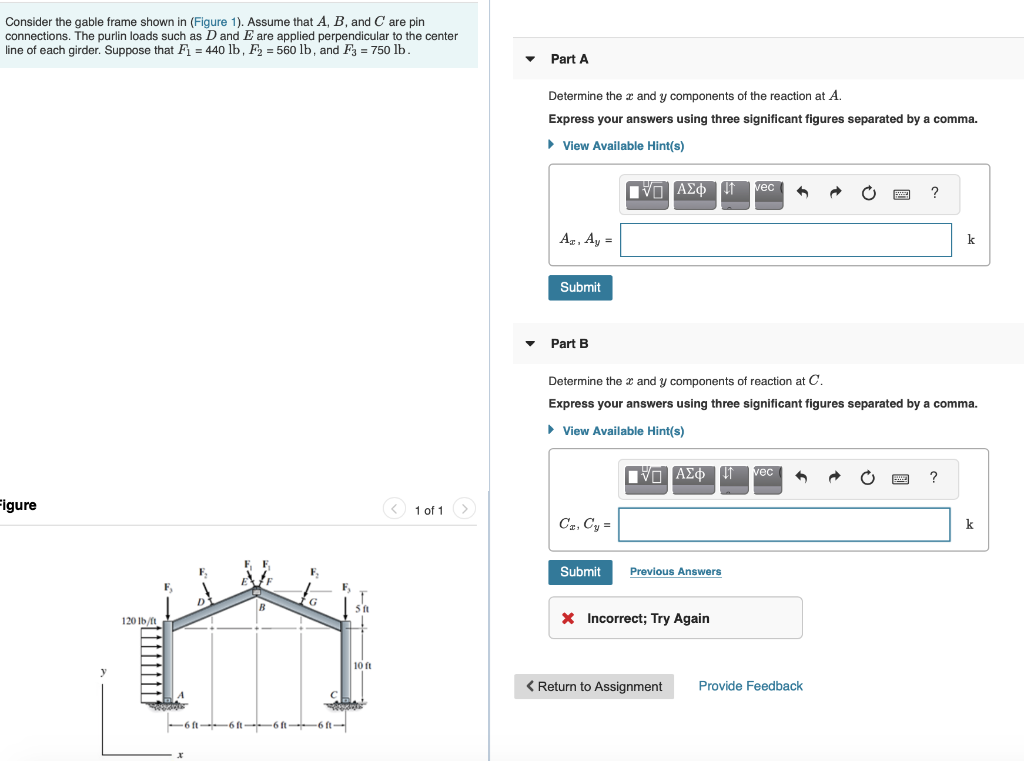# (Solved): Consider The Gable Frame Shown In (Figure 1). Assume That A, B, And C Are Pin Connections. The Purli...Consider the gable frame shown in (Figure 1). Assume that A, B, and C are pin connections. The purlin loads such as D and E are applied perpendicular to the center line of each girder. Suppose that Fi = 440 lb, F2 = 560 lb, and F3 = 750 lb. Part A Determine the x and y components of the reaction at A. Express your answers using three significant figures separated by a comma. View Available Hint(s) VO AÂ¢ * O O ? A., Ay = Submit Part B Determine the 3 and y components of reaction at C Express your answers using three significant figures separated by a comma. View Available Hint(s) Figure < 1 of 1 C.,Cy= Submit Previous Answers X Incorrect; Try Again 10 ft < Return to Assignment Provide Feedback --6--6--6 -6 --

We have an Answer from Expert